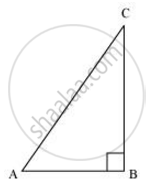Share
Notifications

View all notifications
Books Shortlist
Your shortlist is empty

# Given 15 cot A = 8. Find sin A and sec A - CBSE Class 10 - Mathematics

Login
Create free account

Forgot password?
ConceptTrigonometric Ratios of an Acute Angle of a Right-angled Triangle

#### Question

Given 15 cot A = 8. Find sin A and sec A

#### Solution

Consider a right-angled triangle, right-angled at B.cot A =(AB)/(BC)

= (AB)/(BC)

it is given that

cot A = 8/15

(AB)/(BC)=8/15

Let AB be 8k.Therefore, BC will be 15k, where k is a positive integer.

Applying Pythagoras theorem in ΔABC, we obtain

AC2 = AB2 + BC2

= (8k)2 + (15k)2

= 64k2 + 225k2

= 289k2

AC = 17k

sin A = (15k)/(17k) = 15/17

sec A  = (AC)/(AB) = 17/8

Is there an error in this question or solution?

#### APPEARS IN

NCERT Solution for Mathematics Textbook for Class 10 (2019 to Current)
Chapter 8: Introduction to Trigonometry
Ex.8.10 | Q: 4 | Page no. 181
Solution Given 15 cot A = 8. Find sin A and sec A Concept: Trigonometric Ratios of an Acute Angle of a Right-angled Triangle.
S